Tamil Nadu Board of Secondary EducationHSC Science Class 11

# Tamil Nadu Board Samacheer Kalvi solutions for Class 11th Chemistry Volume 1 and 2 Answers Guide chapter 11 - Fundamentals of Organic Chemistry [Latest edition]

#### Chapters## Solutions for Chapter 11: Fundamentals of Organic Chemistry

Below listed, you can find solutions for Chapter 11 of Tamil Nadu Board of Secondary Education Tamil Nadu Board Samacheer Kalvi for Class 11th Chemistry Volume 1 and 2 Answers Guide.

Evaluation
Evaluation [Pages 153 - 158]

### Tamil Nadu Board Samacheer Kalvi solutions for Class 11th Chemistry Volume 1 and 2 Answers Guide Chapter 11 Fundamentals of Organic Chemistry Evaluation [Pages 153 - 158]

Evaluation | Q I. 1. | Page 153

Select the molecule which has only one π bond.

• CH3 – CH = CH – CH3

• CH3 – CH = CH – CHO

• CH3 – CH = CH – COOH

• All of these

Evaluation | Q I. 2. | Page 153

In the hydrocarbon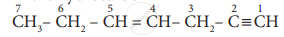the state of hybridisation of carbon 1,2,3,4 and 7 are in the following sequence.

•  sp, sp, sp3, sp2, sp3

• sp2, sp, sp3, sp2, sp3

• sp, sp, sp2, sp, sp3

• none of these

Evaluation | Q I. 3. | Page 153

The general formula for alkadiene is ______.

• CnH2n

• "C"_"n""H"_(2"n" – 1)

• "C"_"n""H"_(2"n" – 2)

• "C"_"n""H"_("n" – 2)

Evaluation | Q I. 4. | Page 154

Structure of the compound whose IUPAC name is 5, 6 – dimethylhept - 2 - ene is

•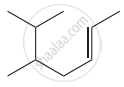•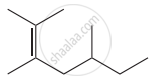•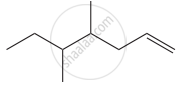• None of these

Evaluation | Q I. 5. | Page 154

The IUPAC name of the compound is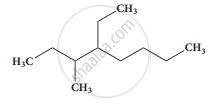• 2,3 - Diemethylheptane

• 3- Methyl -4- ethyloctane

• 5-ethyl -6-methyloctane

• 4-Ethyl -3 - methyloctane

Evaluation | Q I. 6. | Page 154

Which one of the following names does not fit a real name?

• 3 - Methyl - 3 - hexanone

• 4 - Methyl - 3 - hexanone

• 3 - Methyl - 3 hexanol

• 2 - Methyl cyclo hexanone

Evaluation | Q I. 7. | Page 154

The IUPAC name of the compound $\ce{CH3 - CH = CH - C ≡ CH}$ is ______.

• Pent – 4- yn – 2 – ene

• Pent – 3- en – 1- yne

• Pent – 2 – en – 4 – yne

• Pent – 1 yn – 3 – ene

Evaluation | Q I. 8. | Page 154

IUPAC name of $\begin{array}{cc} \phantom{....}\ce{H}\phantom{...}\ce{C4H9}\\ |\phantom{....}|\\\ce{CH3 - C - C - CH3}\\ |\phantom{....}|\\\phantom{.....}\ce{C2H5}\phantom{.}\ce{CH3}\phantom{...}\end{array}$ is

• 3, 4, 4 – Trimethylheptane

• 2 – Ethyl – 3, 3, – dimethyl heptane

• 3, 4, 4 – Trimethyloctane

• 2 – Butyl – 2 – methyl – 3 ethyl – butane

Evaluation | Q I. 9. | Page 154

The IUPAC name of   $\begin{array}{cc} \phantom{.}\ce{CH3}\\|\phantom{..}\\ \ce{H3C - C - CH = C(CH3)2}\\ |\phantom{..}\\\phantom{..}\ce{CH3} \end{array}$ is

• 2,4,4 – Trimethylpent – 2 – ene

• 2,4,4 – Trimethylpent – 3 – ene

• 2,2,4 – Trimethylpent – 3 – ene

• 2,2,4 – Trirnethylpent – 2 – ene

Evaluation | Q I. 10. | Page 154

The IUPAC name of the compound$\begin{array}{cc}\ce{CH3-CH=C-CH2-CH3}\\ |\phantom{..}\\\phantom{...............}\ce{CH2 - CH2 - CH3}\end{array}$ is

• 3 – Ethyl – 2 – hexene

• 3 – Propyl – 3 – hexene

• 4 – Ethyl – 4 – hexene

• 3 – Propyl – 2 – hexene

Evaluation | Q I. 11. | Page 154

The IUPAC name of the compound $\begin{array}{cc}\ce{CH3 - CH - COOH}\\ |\phantom{.....}\\\ce{OH}\phantom{...} \end{array}$ is

• 2 – Hydroxypropionic acid

• 2 – Hydroxy Propanoic acid

• Propan – 2 – ol – 1 – oic acid

• 1 – Carboxyethanol

Evaluation | Q I. 12. | Page 154

The IUPAC name of $\begin{array}{cc} \ce{CH3}\phantom{.....................}\\ \backslash\phantom{....................}\\ \ce{CH - CH - COOH}\\/\phantom{.......}|\phantom{.............}\\ \ce{Br}\phantom{.......}\ce{CH3}\phantom{...........}\\\end{array}$ is

• 2 – Bromo – 3- methyl butanoic acid

• 2 – methyl – 3 bromo butanoic acid

• 2 – methyl – 3 bromo butanoic acid

• 3 – Bromo – 2, 3 – dimethyl propanoic acid

Evaluation | Q I. 13. | Page 154

The structure of isobutyl group in an organic compound is

• CH3 – CH2 – CH2 – CH2

• $\begin{array}{cc}\phantom{..........}\ce{CH3}\\ \phantom{.......}|\\\ce{CH3 - C}\\ \phantom{.......}|\\\phantom{.........}\ce{CH3} \end{array}$

• $\begin{array}{cc}\ce{CH3 - CH - CH2 -}\\ |\phantom{.....}\\\ce{CH3}\phantom{..}\end{array}$

• $\begin{array}{cc}\ce{CH3 - CH - CH2 - CH3}\\ |\phantom{.........}\end{array}$

Evaluation | Q I. 14. | Page 154

The number of stereoisomers of 1, 2 – dihydroxy cyclopentane

• 1

• 2

• 3

• 4

Evaluation | Q I. 15. | Page 155

Which of the following is optically active?

• 3 – Chloropentane

• 2- Chloro propane

• Meso – tartaric acid

• Glucose

Evaluation | Q I. 16. | Page 155

The isomer of ethanol is ______.

• acetaldehyde

• dimethyl ether

• acetone

• methyl carbinol

Evaluation | Q I. 17. | Page 155

How many cyclic and acyclic isomers are possible for the molecular formula C3H6O?

• 4

• 5

• 9

• 10

Evaluation | Q I. 18. | Page 155

Which one of the following shows functional isomerism?

• ethylene

• Propane

• ethanol

• CH2Cl2

Evaluation | Q I. 19. | Page 155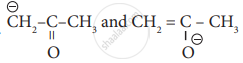are

• resonating structure

• tautomers

• Optical isomers

• Conformers

Evaluation | Q I. 20. | Page 155

Nitrogen detection in an organic compound is carried out by Lassaigne’s test. The blue colour formed is due to the formation of

• Fe3[Fe(CN)6]2

• Fe4 [Fe(CN)6]3

• Fe4[Fe(CN)6]2

• Fe3[Fe(CN)6]3

Evaluation | Q I. 21. | Page 155

Lassaigne’s test for the detection of nitrogen fails in ______.

• $\ce{H2N - CO - NH*NH2*HCl}$

• $\ce{NH2 - NH2*HCl}$

• $\ce{C6H5 - NH - NH2*HCl}$

• $\ce{C6H5CONH2}$

Evaluation | Q I. 22. | Page 155

Connect pair of compounds which give blue colouration/precipitate and white precipitate respectively, when their Lassaigne’s test is separately done.

• NH2 NH2 HCl and ClCH2 – CHO

• NH2 CS NH2 and CH3 – CH2Cl

• NH2 CH2 COOH and NH2 CONH2

• C6H5NH2 and ClCH2 – CHO

Evaluation | Q I. 23. | Page 155

Sodium nitropruside reacts with sulphide ion to give a purple colour due to the formation of ______.

• [Fe (CN)5 NO]3-

• [Fe (NO)5 CN]+

• [Fe (CN)5 NOS]4-

• [Fe (CN)5 NOS]3-

Evaluation | Q I. 24. | Page 155

An organic Compound weighing 0.15g gave on carius estimation, 0.12g of silver bromide. The percentage of bromine in the Compound will be close to ______.

• 46%

• 34%

• 3.4%

• 4.6%

Evaluation | Q I. 25. | Page 155

A sample of 0.5 g of an organic compound was treated according to Kjeldahl’s method. The ammonia evolved was absorbed in 50mL of 0.5 M H2SO4 The remaining acid after neutralization by ammonia consumed 80 mL of 0.5 M NaOH. The percentage of nitrogen in the organic compound is.

• 14%

• 28%

• 42%

• 56%

Evaluation | Q I. 26. | Page 156

In an organic compound, phosphorus is estimated as ______.

• Mg2P2O7

• Mg3(PO4)2

• H3PO4

• P2O 5

Evaluation | Q I. 27. | Page 156

Ortho and para – nitro phenol can be separated by ______.

• azeotropic distillation

• destructive distillation

• steam distillation

• cannot be separated

Evaluation | Q I. 28. | Page 156

The purity of an organic compound is determined by ______.

• Chromatography

• Crystallisation

• melting or boiling point

• both Chromatography and melting or boiling point

Evaluation | Q I. 29. | Page 156

A liquid which decomposes at its boiling point can be purified by ______.

• distillation at atmospheric pressure

• distillation under reduced pressure

• fractional distillation

• steam distillation

Evaluation | Q I. 30. | Page 156

Assertion: $\begin{array}{cc} \ce{CH - C = CH - COOH}\\|\phantom{.}\\ \phantom{........}\ce{COOC2H5}\end{array}$

Reason: The principal functional group gets lowest number followed by double bond (or) triple bond.

• both the assertion and reason are true and the reason is the correct explanation of assertion.

• both assertion and reason are true and the reason is not the correct explanation of assertion.

• assertion is true but reason is false.

• both the assertion and reason are false

#### Write brief answer to the following questions.

Evaluation | Q II. 1. | Page 156

Give the general characteristics of organic compounds.

Evaluation | Q II. 2. | Page 156

Describe the classification of organic compounds based on their structure.

Evaluation | Q II. 3. | Page 156

Write a note on homologous series.

Evaluation | Q II. 4. (a) | Page 156

Identify the functional group in the following compound.

Acetaldehyde

Evaluation | Q II. 4. (b) | Page 156

Identify the functional group in the following compound.

Oxalic acid

Evaluation | Q II. 4. (c) | Page 156

Identify the functional group in the following compound.

Di methyl ether

Evaluation | Q II. 4. (d) | Page 156

Identify the functional group in the following compound.

Methylamine

Evaluation | Q II. 4. | Page 156

What is meant by a functional group?

Evaluation | Q II. 5. (a) | Page 156

Give the general formula for the following class of organic compound.

Aliphatic monohydric alcohol

Evaluation | Q II. 5. (b) | Page 156

Give the general formula for the following class of organic compound.

Aliphatic ketones

Evaluation | Q II. 5. (c) | Page 156

Give the general formula for the following class of organic compound.

Aliphatic amines

Evaluation | Q II. 6. | Page 156

Write the molecular formula of the first six members of homologous series of nitro – alkanes.

Evaluation | Q II. 7. | Page 156

Write the molecular formula and possible structural formula of the first four members of homologous series of carboxylic acids.

Evaluation | Q II. 8. (i) | Page 156

Give the IUPAC names of the following compound.

$\ce{(CH3)2 CH–CH2 –CH(CH3 )–CH(CH3)2}$

Evaluation | Q II. 8. (ii) | Page 156

Give the IUPAC names of the following compound.

$\begin{array}{cc} \ce{CH3 - CH - CH - CH3}\\ \phantom{...}|\phantom{......}|\phantom{.....}\\ \ce{CH3}\phantom{...}\ce{Br}\phantom{.} \end{array}$

Evaluation | Q II. 8. (iii) | Page 156

Give the IUPAC names of the following compound.

$\ce{CH3 - O - CH3}$

Evaluation | Q II. 8. (iv) | Page 156

Give the IUPAC names of the following compound.

$\begin{array}{cc}\ce{CH3 - CH2 - CH - CHO}\\ \phantom{.....}|\\\phantom{.......}\ce{OH} \end{array}$

Evaluation | Q II. 8. (v) | Page 156

Give the IUPAC names of the following compound.

$\ce{CH2 = CH - CH = CH2}$

Evaluation | Q II. 8. (vi) | Page 156

Give the IUPAC names of the following compound.

$\begin{array}{cc}\ce{CH3 - C ≡ C - CH - CH3}\\ \phantom{........}|\\\phantom{.........}\ce{Cl} \end{array}$

Evaluation | Q II. 8. (vii) | Page 157

Give the IUPAC names of the following compound.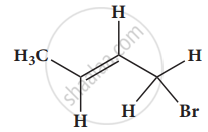Evaluation | Q II. 8. (viii) | Page 157

Give the IUPAC names of the following compound.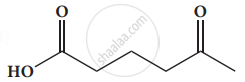Evaluation | Q II. 8. (ix) | Page 157

Give the IUPAC names of the following compound.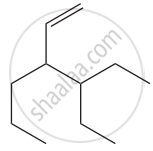Evaluation | Q II. 8. (x) | Page 157

Give the IUPAC names of the following compound.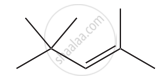Evaluation | Q Evaluation | Page 157

Give the IUPAC names of the following compound.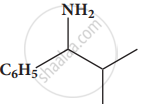Evaluation | Q II. 8. (xii) | Page 157

Give the IUPAC names of the following compound.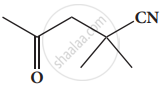Evaluation | Q II. 8. (xiii) | Page 157

Give the IUPAC names of the following compound.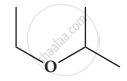Evaluation | Q II. 8. (xiv) | Page 157

Give the IUPAC names of the following compound.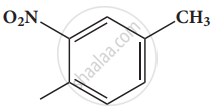Evaluation | Q II. 8. (xv) | Page 157

Give the IUPAC names of the following compound.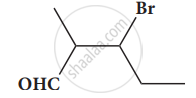Evaluation | Q II. 9. (i) | Page 157

Give the structure for the following compound.

3 – ethyl – 2 methyl – 1 – pentene

Evaluation | Q II. 9. (ii) | Page 157

Give the structure for the following compound.

1,3,5- Trimethyl cyclohex - 1 -ene

Evaluation | Q II. 9. (iii) | Page 157

Give the structure for the following compound.

tertiary butyl iodide

Evaluation | Q II. 9. (iv) | Page 157

Give the structure for the following compound.

3 - Chlorobutanal

Evaluation | Q II. 9. (v) | Page 157

Give the structure for the following compound.

3 - Chlorobutanol

Evaluation | Q II. 9. (vi) | Page 157

Give the structure for the following compound.

2 - Chloro - 2- methyl propane

Evaluation | Q II. 9. (vii) | Page 157

Give the structure for the following compound.

2,2-dimethyl-1-chloropropane

Evaluation | Q II. 9. (viii) | Page 157

Give the structure for the following compound.

3 - methylbut -1- ene

Evaluation | Q II. 9. (ix) | Page 157

Give the structure for the following compound.

Butan - 2, 2 - diol

Evaluation | Q II. 9. (x) | Page 157

Give the structure for the following compound.

Octane - 1,3- diene

Evaluation | Q II. 9. (xi) | Page 157

Give the structure for the following compound.

1,3- Dimethylcyclohexane

Evaluation | Q II. 9. (xii) | Page 157

Give the structure for the following compound.

3-Chlorobut - 1 - ene

Evaluation | Q II. 9. (xiii) | Page 157

Give the structure for the following compound.

3 - methylbutan - 2 - ol

Evaluation | Q II. 9. (xiv) | Page 157

Give the structure for the following compound.

acetaldehyde

Evaluation | Q II. 10. | Page 157

Describe the reactions involved in the detection of nitrogen in an organic compound by Lassaigne method.

Evaluation | Q II. 11. | Page 158

Give the principle involved in the estimation of halogen in an organic compound by Carius method.

Evaluation | Q II. 12. i) | Page 158

Give a brief description of the principles of Fractional distillation.

Evaluation | Q II. 12. ii) | Page 158

Give a brief description of the principle of column Chromatography.

Evaluation | Q II. 13. | Page 158

Explain paper chromatography.

Evaluation | Q II. 14. | Page 158

Explain various types of constitutional isomerism (structural isomerism) in organic compounds.

Evaluation | Q II. 15. | Page 158

Describe optical isomerism with a suitable example.

Evaluation | Q II. 15. | Page 158

Describe optical isomerism with a suitable example.

Evaluation | Q II. 16. | Page 158

Briefly explain geametrical isomerism in alkene by considering 2-butene as an example.

Evaluation | Q II. 17. | Page 158

0.30 g of a substance gives 0.88 g of carbon dioxide and 0.54 g of water calculate the percentage of carbon and hydrogen in it.

Evaluation | Q II. 18. | Page 158

The ammonia evolved form 0.20 g of an organic compound by Kjeldahl method neutralized 15 ml of N/20 sulphuric acid solution. Calculate the percentage of Nitrogen.

Evaluation | Q II. 19. | Page 158

0.32 g of an organic compound, after heating with fuming nitric acid and barium nitrate crystals is a sealed tube game 0.466 g of barium sulphate. Determine the percentage of sulphur in the compound.

Evaluation | Q II. 20. | Page 158

0.24g of an organic compound gave 0.287 g of silver chloride in the carius method. Calculate the percentage of chlorine in the compound.

Evaluation | Q II. 21 | Page 158

In the estimation of nitrogen present in an organic compound by Dumas method 0.35 g yielded 20.7 mL of nitrogen at 15° C and 760 mm pressure. Calculate the percentage of nitrogen in the compound.

## Solutions for Chapter 11: Fundamentals of Organic Chemistry

Evaluation## Tamil Nadu Board Samacheer Kalvi solutions for Class 11th Chemistry Volume 1 and 2 Answers Guide chapter 11 - Fundamentals of Organic Chemistry

Shaalaa.com has the Tamil Nadu Board of Secondary Education Mathematics Class 11th Chemistry Volume 1 and 2 Answers Guide Tamil Nadu Board of Secondary Education solutions in a manner that help students grasp basic concepts better and faster. The detailed, step-by-step solutions will help you understand the concepts better and clarify any confusion. Tamil Nadu Board Samacheer Kalvi solutions for Mathematics Class 11th Chemistry Volume 1 and 2 Answers Guide Tamil Nadu Board of Secondary Education 11 (Fundamentals of Organic Chemistry) include all questions with answers and detailed explanations. This will clear students' doubts about questions and improve their application skills while preparing for board exams.

Further, we at Shaalaa.com provide such solutions so students can prepare for written exams. Tamil Nadu Board Samacheer Kalvi textbook solutions can be a core help for self-study and provide excellent self-help guidance for students.

Concepts covered in Class 11th Chemistry Volume 1 and 2 Answers Guide chapter 11 Fundamentals of Organic Chemistry are Introduction to Fundamentals of Organic Chemistry, Classification of Organic Compounds, Nomenclature of Organic Compounds, Structural Representation of Organic Compounds, Isomerism in Organic Compounds, Detection of Elements in Organic Compounds, Estimation of Elements, Introduction of Methods of Purification of Organic Compounds.

Using Tamil Nadu Board Samacheer Kalvi Class 11th Chemistry Volume 1 and 2 Answers Guide solutions Fundamentals of Organic Chemistry exercise by students is an easy way to prepare for the exams, as they involve solutions arranged chapter-wise and also page-wise. The questions involved in Tamil Nadu Board Samacheer Kalvi Solutions are essential questions that can be asked in the final exam. Maximum Tamil Nadu Board of Secondary Education Class 11th Chemistry Volume 1 and 2 Answers Guide students prefer Tamil Nadu Board Samacheer Kalvi Textbook Solutions to score more in exams.

Get the free view of Chapter 11, Fundamentals of Organic Chemistry Class 11th Chemistry Volume 1 and 2 Answers Guide additional questions for Mathematics Class 11th Chemistry Volume 1 and 2 Answers Guide Tamil Nadu Board of Secondary Education, and you can use Shaalaa.com to keep it handy for your exam preparation.